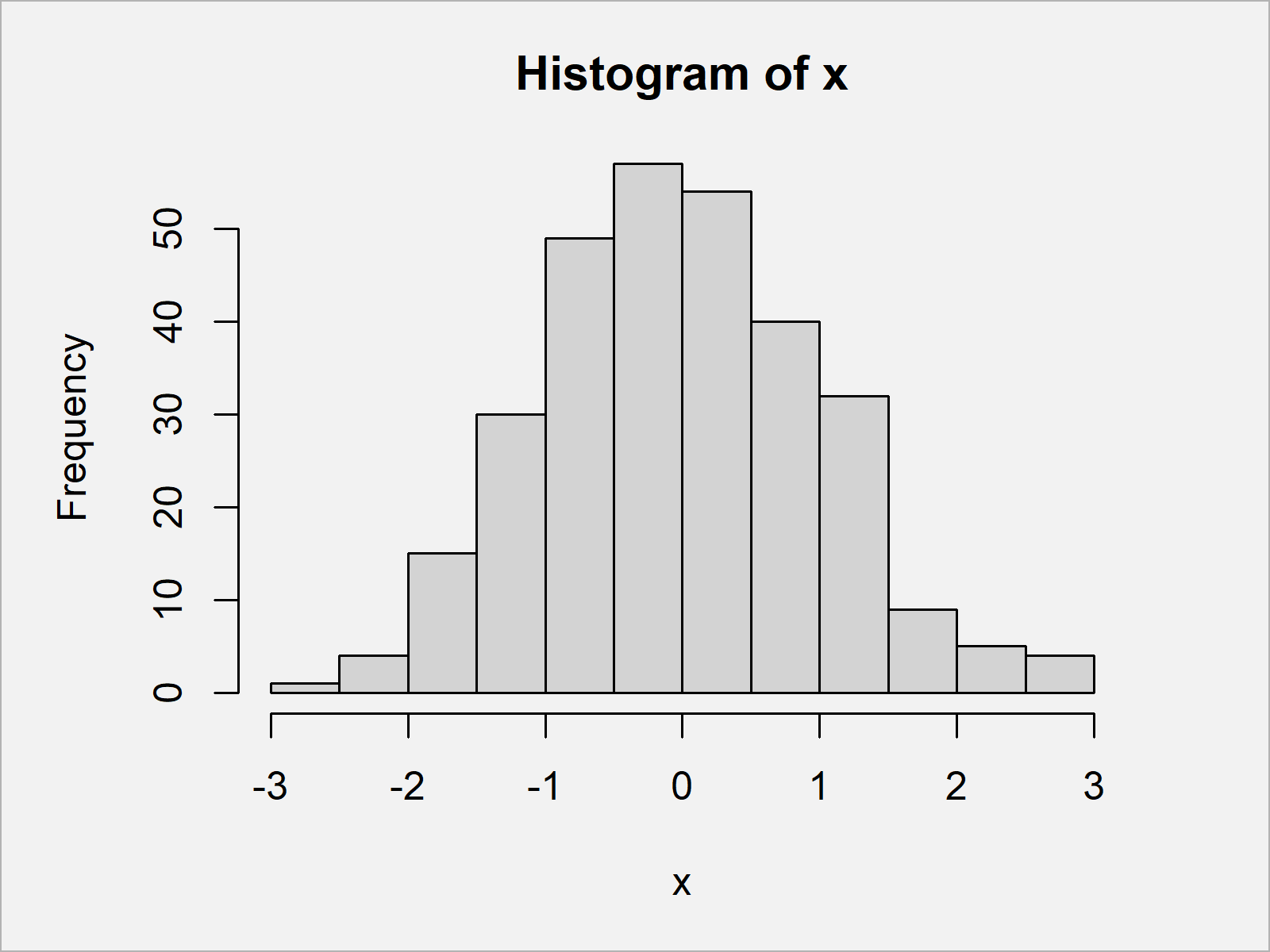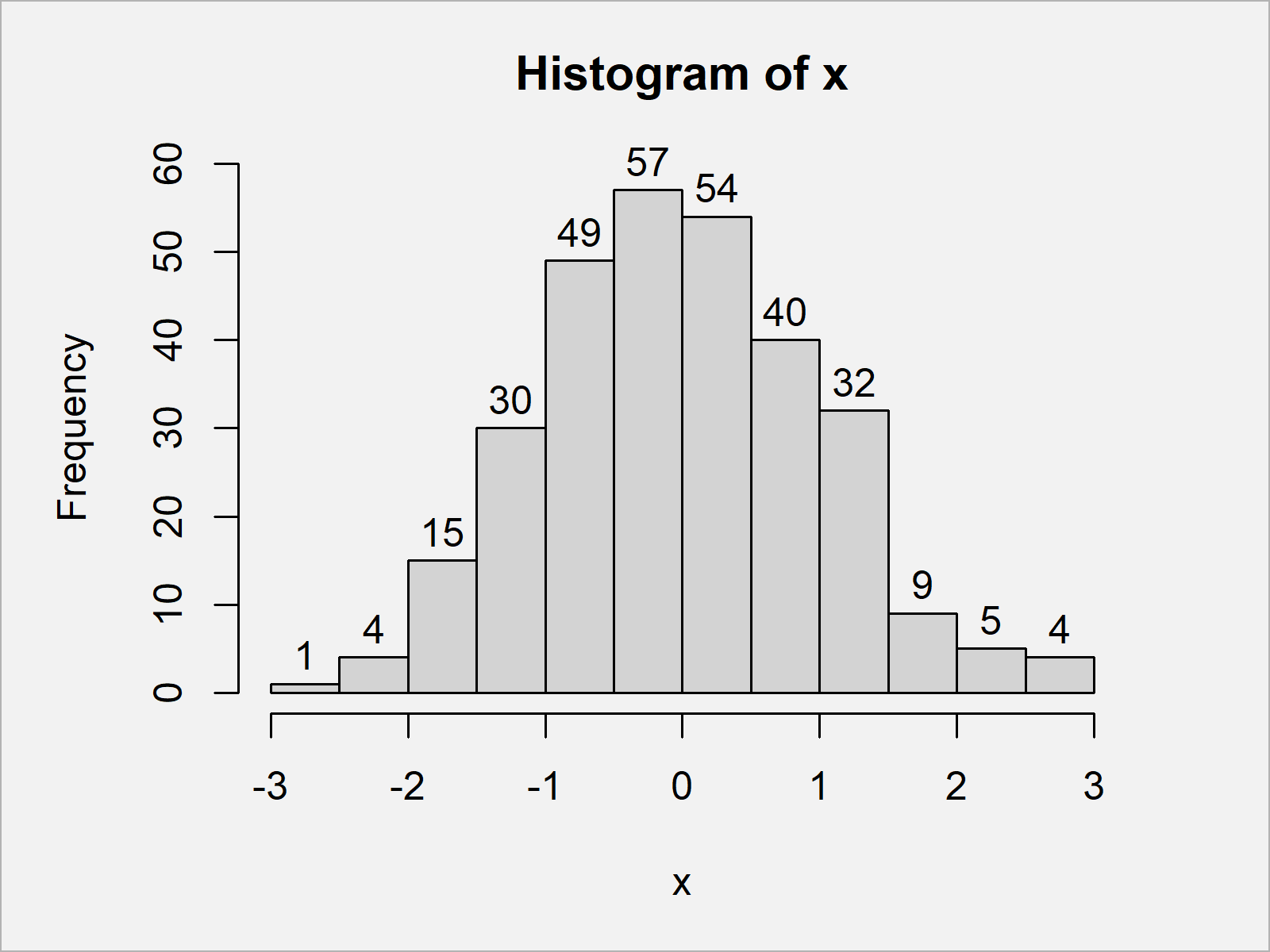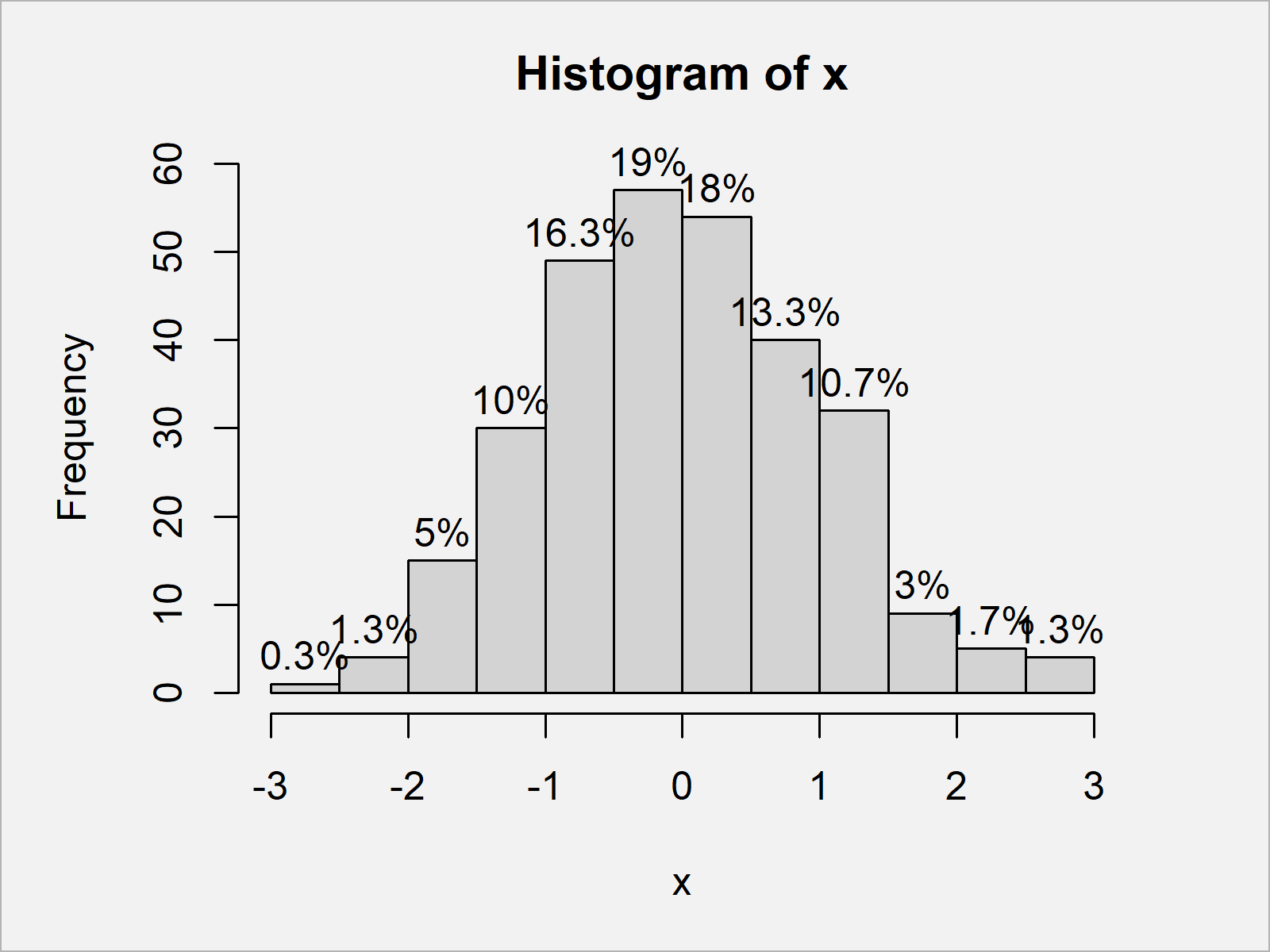# Add Count & Percentage Labels on Top of Histogram Bars in R (2 Examples)

This article shows how to draw frequencies and percentage points above each bar of a histogram in R.

Sound good? Let’s get started.

## Creation of Example Data

Let’s first construct some example data:

```set.seed(92365)                     # Create example data
x <- rnorm(100)
head(x)                             # Print first values of data
#  -1.94404137 -0.28602202  1.90124067  0.05188518  0.25174766  0.23888625```

The previous output of the RStudio console reveals that the example data is a numeric vector containing randomly distributed values.

Now, we can create a graphic of our data as shown below:

`hist(x)                             # Draw default histogram`As visualized in Figure 1, we have created a histogram using Base R by executing the previous R programming syntax. This histogram does not show any labels on top of the bars yet.

## Example 1: Add Count Labels on Top of Histogram Bars

In this example, I’ll show how to draw frequency counts on top of the bars of our histogram.

For this, we simply have to specify the labels argument within the hist function to be equal to TRUE:

```hist(x,                             # Add count labels
labels = TRUE)```In Figure 2 it is shown that we have plotted a Base R histogram with frequency count labels on top of the bars.

## Example 2: Add Percentage Labels on Top of Histogram Bars

In this example, I’ll illustrate how to display the labels on top of a histogram in percentage points.

Have a look at the following R code:

```hist(x,                             # Add percentage labels
labels = paste0(round(hist(x, plot = FALSE)\$counts / length(x) * 100, 1), "%"))```In Figure 3 it is shown that we have created a histogram with percentage points over the bars.

The R syntax of this example might look a bit complicated. However, you can just copy and paste the R code shown here and use it for your own application.

## Video, Further Resources & Summary

Have a look at the following video of my YouTube channel. In the video, I’m showing the R syntax of this article in RStudio:

Please accept YouTube cookies to play this video. By accepting you will be accessing content from YouTube, a service provided by an external third party.If you accept this notice, your choice will be saved and the page will refresh.

Furthermore, you might read the other tutorials of this website:

In this R tutorial you have learned how to show counts and percentages in histograms. Let me know in the comments, in case you have additional questions.

Subscribe to the Statistics Globe Newsletter

•